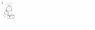# Tension in pulley systems

• BMcC

#### BMcC

Give the correct tension, T, in the marked rope for each situation. The rope is held so the system is stationary. All ropes and pulleys are massless and frictionless.

A) T = W
B) T = W/2
C) T = W/3
D) T = 2W
E) T = 3W/2
F) T = 2W/3
G) T = W/7
H) T = W/5
I) T = 2W/7
J) T = 2W/5

1) I figure that the two pulleys holding the weight W each have a tension of W/2, making the first B) T = W/2

2) The three pulleys holding the weight W each have a tension W/3, and the pulley holding the two pulleys connected to the weight must have a tension of 2W/3, making the second F) T = 2W/3

3) The three pulleys holding weight 2W each have a tension 2W/3, and the pulley ropes holding those pulleys each have a tension of 2W/3, divided by 2 = 2W/6 = W/3. This makes T = W/3

4) I'm not quite sure how to do this one

Hi BMcC

Welcome to PhysicsForums!

Instead of the guessing game,first understand the concepts ,and take one step at a time.Get in the habit of making Free Body Diagram(FBD). Consider the two pulleys separately ,identify and clearly draw all the forces acting on them.

Remember tension in a massless string is same throughout its length.

Look at the figure I have attached.Consider the lower left pulley.

The forces acting on it are tension(2-green upwards),T downwards and tension (1-Blue downwards).

The tension throughout the string over the pulley is same .Since the force exerted on the left part of the string is T ,the tension in the right part(1-Blue) is also T .

Next consider the top pulley.The tension in the left part of the string(2-green) should be 2T .Why?

Because since the lower left pulley is massless ,the net force on it should be zero.It is being pulled down by a force 2T(T downwards from left end and T downwards from right end) .So tension in the left part of the string((2-green) should be 2T.

This 2T gets transmitted throughout the string over the top pulley .So tension on the right part of the string (3-Red)over the top pulley is 2T .

Now can you figure out what is the upward force on weight W ?

#### Attachments

•first fig.jpg
3.3 KB · Views: 900
Last edited:
There's a way to analyze these systems to eliminate the guesswork.

Isolate each sheave and make a free body diagram.

So the upward force on weight W for #1 would be 3T?

So the upward force on weight W for #1 would be 3T?

Yes...

•1 person
Then in 2), would the upward force on weight also be 3T? from the T/2 + T/2 on the small pulleys, then +2T from the long rope on the far right?

Then in 2), would the upward force on weight also be 3T? from the T/2 + T/2 on the small pulleys, then +2T from the long rope on the far right?

Yes...Very Good :)